If you have a related question, please click the "Ask a related question" button in the top right corner. The newly created question will be automatically linked to this question.

# DAC8822: Designing DAC8822 for sine wave

Part Number: DAC8822
Other Parts Discussed in Thread: OPA2277, OPA227, THS4031

Hi Team,
I want to generate a sine wave of frequencies 10KHz and 250KHz from DAC8822.
For a brief reading of the datasheet, I've needed some clarifications and hoping you guys can solve it.
The DAC is communication with a 3.3V logic controller. The minimum logic input voltage when driven from a +5V is 2.4V.
1). Which are the logic output pins?.
3). I want a +/-14V bipolar sine wave from the DAC, so what should be the value of Vref?.
4).In the table below, if I understand it correctly,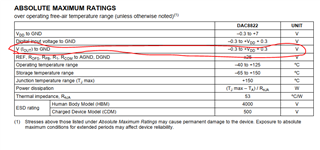The output of the DAC would be only 0 to 5V for VDD=5V. Or am I wrong?.
5). The output of the DAC is fed to a 4th order LPF,

• Hi,

1. What do you mean by logic output pins ?

Here the DAC has all logic inputs, no logic output pins. With 3.3V, logic high will be 2.1V and logic low will be 0.65V

In an mDAC like DAC8822, you can have either 2 quadrant or 4 quadrant operation.

A system only including the MDAC and transimpedance stage, highlighted in below figure ,would be referred to as a two-quadrant configuration because the output is only able to swing positive or negative by changing the reference voltage polarity.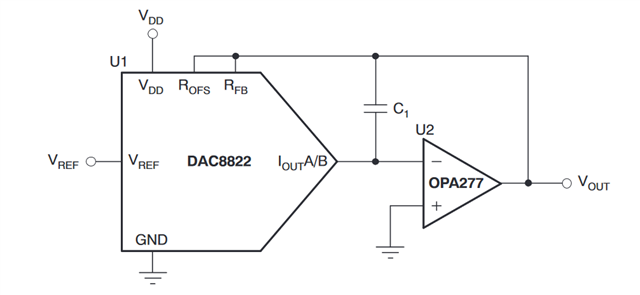The four-quadrant system is capable of positive or negative output voltages by changing either the reference voltage or by changing DAC codes.

In DAC8822's case the below configuration gives 4 quadrant operation.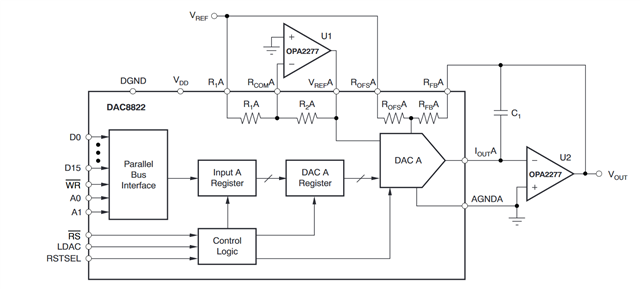Graphically we can represent both modes of operation as follows.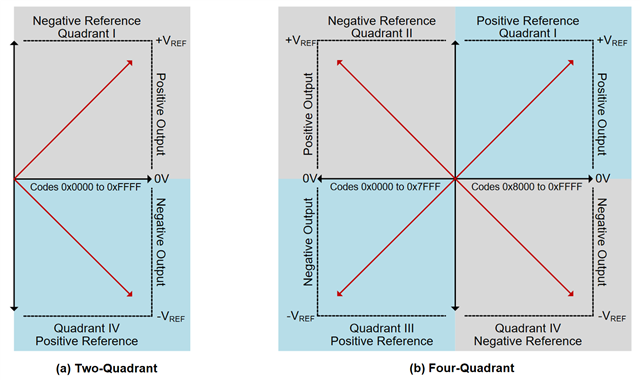3. DAC8822 supports reference voltages upto 18V. In your case you can use 14V as reference. DAC output will vary from -14V to +14V if you use 4 quadrant operation.Since 14V is not standard reference, you can use 10V as Vref and use an additional op amp stage to gain it up. You can have this gain stage in LPF itself.

4. Thats the compliance voltage of Iout pin, DAC8822 output is current, not voltage.

5. You can have LPF after transimpedance stage.

Hope this clarifies all your queries. Let me know if you need more help.

Regards,

AK

• Hi AK,
By "logic output pin" I mean any output pin of DAC to the controller and you made it clear. Thanks.
I need an output signal +/-14V, so I think 4 quadrant mode is enough with a reference voltage of +10V like you said.

• Hi Shibin,

Regards,

AK

• Hi Akhilesh,
I hope I can clarify my doubts in this resolved forum.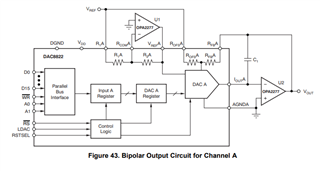Why we need an external reference amplifier U1 in the above setup?.
How to drive U1 or what are its voltage rail requirements?.
My Vref is set to +10V.
I want to generate a sine wave of +/-10V with a frequency 250KHz. But the slew rate of the op-amp OPA2277 seems very less (0.8V/uS). Will this create any problem?.

• Hi Shibin,

Please note that the polarity of the full-scale output (IOUT) is the inverse of the input reference voltage at VREF. So with U1 we are basically inverting the reference to DAC as -10V ( VREF  = 10V). With VREF given to one of the resistors (ROFS_A), we are going to generate the required -10V to 10V from DAC as code moves from 0 to full code.

U1 rails depends on the reference voltage. If its 10V, I suggest +/-12V min and choose OPA227.

If you want to generate 250Khz sine wave with +/-10V amplitude, min slew rate requirement can be calculated using the following equation.

Slew Rate  = 2* Pi * Freq * Amplitude (Peak to Peak)

In your case min requirement comes out to be approx 32V/uS. Please choose an Op amp with min gain bandwdith product of 25Mhz (min) and Slew Rate as 32V/uS (min)

Have a look at the Op amp portfolio and choose the one which satify this requirement. I can recommend THS4031.

https://www.ti.com/product/THS4031

Its better to open a new thread for new questions ( It helps us to track the thread better)

Regards,

AK